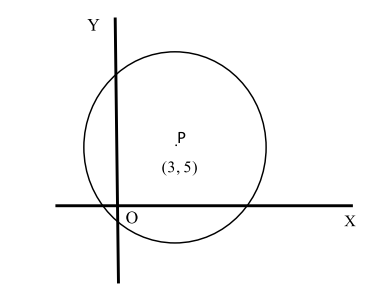# Free SAT Test Questions

Question 1 of 1
ID: SAT-AM-10
Section: Math - Additional Topics in Math
Topic: Coordinate Geometry
Difficulty level: Hard

(Practice Mode: Single selected Question » Back to Overview)

Which of the following can be a possible equation of the circle shown in the following diagram with P as the center of the circle and O is the origin of system of coordinate axes? (With calculator)A${(x-3)}^2+{(y-5)}^2=30$
B${(x-3)}^2+{(y-5)}^2=25$
C${(x-3)}^2+{(y-5)}^2=40$
D${(x-3)}^2+{(y-5)}^2=34$

#### Finding the Right Time to Take the SAT

Read on the optimal timings to sit for the SAT that will give you a competitive edge. With the SAT only being offered a few times every year, it is critical to find the right time to take your exam.